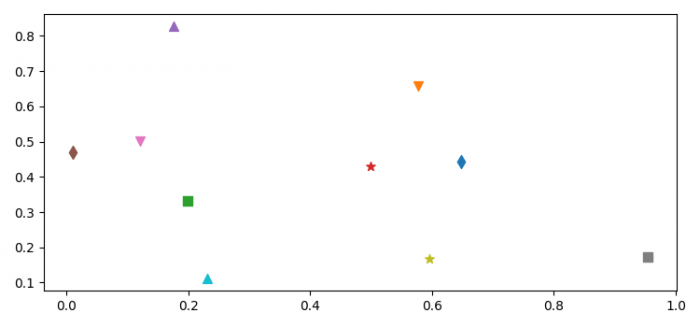# How to use different markers for different points in a Pylab scatter plot(Matplotlib)?

MatplotlibPythonData Visualization

To use different markers for different points in a Pylab (Pyplot) scatter plot, we can use the following steps −

• Set the figure size and adjust the padding between and around the subplots.
• Initialize a variable, N, for number of sample data.
• Create x and y random data points.
• Make a list of markers.
• Zip the x, y and markers.
• Iterate the zipper objects and plot the data points with different markers.
• To display the figure, use show() method.

## Example

import numpy as np
from matplotlib import pyplot as plt

plt.rcParams["figure.figsize"] = [7.50, 3.50]
plt.rcParams["figure.autolayout"] = True

N = 10
x = np.random.rand(N)
y = np.random.rand(N)

markers = ["d", "v", "s", "*", "^", "d", "v", "s", "*", "^"]

for xp, yp, m in zip(x, y, markers):
plt.scatter(xp, yp, marker=m, s=50)

plt.show()

## Output